# Using prior distributions with 'ratematrix'

Here we are going to set uninformative and informative priors for each of the parameters of the model. Please note that model estimation is based on Markov-chain Monte Carlo (MCMC), so the analyses described here might take some time to run.

## Priors on the root value

The 'ratematrix' package estimates the root value and the evolutionary rate matrix (R) for a multivariate Brownian-motion model (mvBM) with single or multiple rate regimes fitted to the same phylogenetic tree. The mvBM model (and also the single trait BM model) have the behavior that trait values estimated for the nodes approach the mean of the tip data as we traverse the nodes of the tree from the tips to the root. This happens because BM models have a fixed mean and the variance increases proportional to time. As a result, we known a priori that the estimated root is not going to be outside the range of the observed tip values and will be likely close to the mean of the tip data. Such information about the statistical behavior of the model, even if not directly related to biology, can help us design prior distributions to the Markov-chain Monte Carlo (MCMC) analysis.

It is important to note that this behavior is expected for the estimated root value regardless of the true root value. The BM model, as well as many other phylogenetic comparative models of trait evolution, requires information at the nodes of the phylogeny for a more accurate estimate of the root value. Such information can come from fossil data, for example.

``````library( ratematrix )
library( geiger )
``````
``````## Loading required package: ape
``````

Simulate some data. Here we are using a phylogeny of 100 tips and two traits. However, it is straightforward to extend this analysis to more traits.

``````phy <- sim.bdtree(b=1, d=0, stop="taxa", n=100)
R <- rbind(c(0.5, 0.2),
c(0.2, 0.5) )
dat <- simRatematrix(tree=phy, vcv=R, anc=c(5,10))
``````

We will run two separated analysis using this data. One with a uniform prior for the root values and another with a normally distributed prior (informative prior) centered far from the true parameter value for the model. The objective of this exercise is to show how to set your own custom prior distribution and how the model will behave if this prior is misguided.

### Uniform prior on the root

First we need to set the prior. Although a uniform prior can be easily set using built-in options of the function `ratematrixMCMC`, here we 'manually' define the prior as a worked example using the function `makePrior()` .

``````## Create a matrix the bounds for the uniform prior.
## Here will use the max and min of the tip data as bounds.
par.mu <- rbind( ceiling( range(dat[,1]) ), ceiling( range(dat[,2]) ) )
## Using a lognormal prior on the standard deviations, with log(mean)=0 and log(sd)=1.5.
par.sd <- c(0, 1.5)
## Prior on the covariance matrix is set to the default:
##          marginally uniform on the covariances.
unif.prior <- makePrior(r = 2, p = 1, den.mu = "unif", par.mu = par.mu, den.sd = "lnorm"
, par.sd = par.sd)
``````

After defining our prior we can take samples from it and evaluate the distribution by plotting the samples.

``````samples <- samplePrior(n = 1000, prior = unif.prior)
## Plot the prior samples between -10 and 10.
plotRatematrix(samples, set.xlim = c(-10,10))
``````
``````## Plotting a single regime.
``````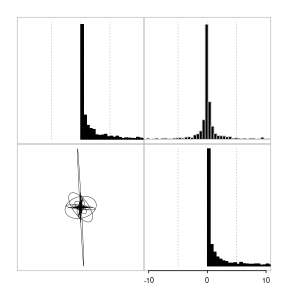Now we are ready to run the MCMC analysis using the prior we created. Here I slightly increased the frequency that the chain is sampling the root values. Note that both the sampling frequencies for each parameter of the model (`prop`) and the width of the proposals (`w_sd` and `w_mu`) are parameters that can (and should!!) be tweaked by the user to maximize the efficiency of the sampler.

The `ratematrixMCMC` function will write files to the working directory. The output is a handle object that stores the information of the MCMC run. The handle object (here named `handle.unif.root`) will be required by other functions.

``````handle.unif.root <- ratematrixMCMC(data=dat, phy=phy, prior=unif.prior
, start="prior_sample", gen=200000
, outname="uniform_root_prior", dir=tempdir() )
``````

The next code block will read the MCMC output from the files and make a fast convergence analysis.

``````## post.unif.root <- readMCMC(handle=handle.unif.root)
## Check the posterior object:
## post.unif.root
## checkConvergence(post.unif.root)
``````

This convergence analysis is based only on a single chain. The output table with TRUE/FALSE values are the result of the Heidel test (see package `coda` for more information). If provided with multiple chains, `checkConvergence` will compute the Gelman and Rubin (1992) potential reduction factor index, which is a much more robust test for convergence. The function `logAnalyzer` will make traceplots for the run.

Now we can plot the prior used for this analysis. Note that the prior on the root values is flat within the specified range. Of course, the histogram is based on samples from the prior distribution, so the result is not completely flat.

``````plotPrior(handle=handle.unif.root, root=TRUE)
``````
``````## Plotting the prior distribution.
## IMPORTANT NOTE: Pay attention to the scale of the rates when comparing the posterior and prior distribution plots!!.
``````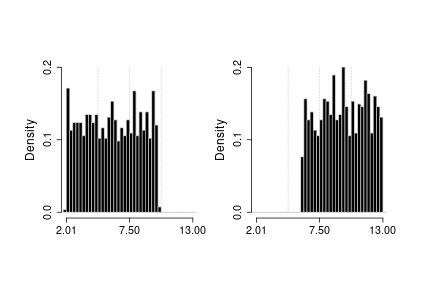In the same way, it is simple to make a quick check on the prior distribution for the evolutionary rate matrix (R)

``````plotPrior(handle=handle.unif.root, set.xlim=c(-10,10))
``````
``````## Plotting the prior distribution.
## IMPORTANT NOTE: Pay attention to the scale of the rates when comparing the posterior and prior distribution plots!!.
## Plotting a single regime.
``````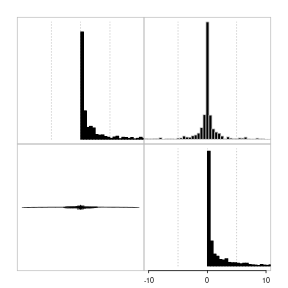Here we plot the posterior distribution for the vector of root values. Vertical red lines show the true parameter value used to simulate the data.

``````plotRootValue(post.unif.root, vline.values = c(5,10), vline.color = c("red","red"))
``````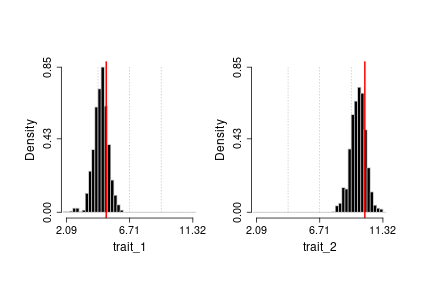This plot makes it clear that the posterior distribution includes the true parameter value within its 95% highest posterior density (HPD) interval. This result is expected, since we simulated data under the model.

### Informative prior on the root

What if the prior on the root value is informative? The two most common reasons to implement informative priors are; a) use of external information such as trait values for the nodes (e.g., fossil data) or knowledge of the underlying genetic architecture of the traits under study, or b) to bond parameter values to a reasonable region of the parameter space when faced with difficulty in convergence. The first case is the most clear use of prior distributions. That is to inform our analysis based on extra information about the problem. The second case is more technical. The main point here is that when some of the parameters of a model are hard to be estimated or when there are not enough information in the data (maybe both!), the likelihood surface for the model might become flat on some regions and/or show some ridges. When the sampler gets stuck in some of these ridges the parameter values can become unrealistic (i.e., super large or small numbers with limited biological interpretation). In this case, even a weak prior distribution favoring reasonable parameter values can help the chain reach convergence. A useful analogy for this problem is the use of bounded maximum likelihood searches (see option “L-BFGS-B” in function `optim`). We suggest checking work by Uyeda and Harmon (A Novel Bayesian Method for Inferring and Interpreting the Dynamics of Adaptive Landscapes from Phylogenetic Comparative Data, 2014, Systematic Biology) for an interesting example on the use of informative priors.

Here we are going to intentionally set a prior centered on values away from the true parameter values. This is an extreme example that will show the impact of the prior distribution on the posterior distribution. Then we will explore the effect that this has on the analysis results.

First we generate the prior distribution with steps similar to what we did before. Then we run analysis and gather results.

``````## Generate a matrix with the parameters for a normal distribution for the root values.
## Root_1: mean 0 and sd 1 and Root_2: mean 15 and sd 2.
par.mu <- rbind( c(0, 1), c(15, 2) )
## Using a lognormal prior on the standard deviations, with log(mean)=0 and log(sd)=1.5.
par.sd <- c(0, 1.5)
## Prior on the covariance matrix is set to the default:
##       marginally uniform on the covariances.
norm.prior <- makePrior(r = 2, p = 1, den.mu = "norm", par.mu = par.mu, den.sd = "lnorm"
, par.sd = par.sd)
``````
``````handle.norm.root <- ratematrixMCMC(data=dat, phy=phy, prior=norm.prior
, start="prior_sample", gen=200000
, outname="normal_root_prior", prop=c(0.1,0.45,0.45)
, dir=tempdir() )
``````
``````## post.norm.root <- readMCMC(handle=handle.norm.root)
checkConvergence(post.norm.root)
``````
``````## \$heidel
##       root matrix
## stest TRUE   TRUE
## htest TRUE   TRUE
##
## \$ess
##       root_1       root_2 matrix_cel_1 matrix_cel_2 matrix_cel_3 matrix_cel_4
##     165.2597     103.7021     393.1583     430.8257     430.8257     455.0331
``````

The Heidel test suggests the single chain reached convergence. Now we can make plots of the prior and posterior distributions.

``````plotPrior(handle=handle.norm.root, root=TRUE)
``````
``````## Plotting the prior distribution.
## IMPORTANT NOTE: Pay attention to the scale of the rates when comparing the posterior and prior distribution plots!!.
``````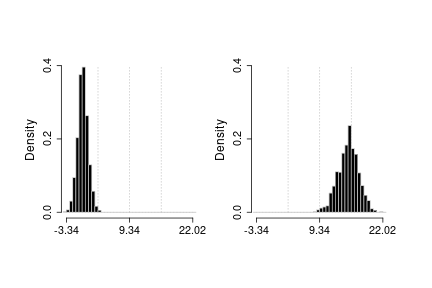``````plotRootValue(post.norm.root, vline.values = c(5,10), vline.color = c("red","red"))
``````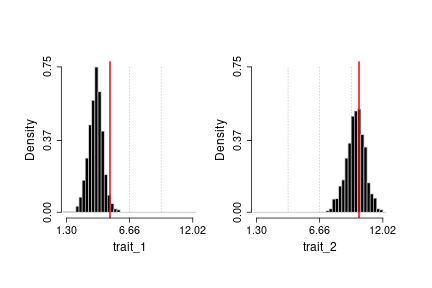Note how the true parameter value for root of trait_1 (red line) is away from the prior distributions whereas the root value for trait_2 is. Now compare the plot of the prior distribution with the plot for the posterior. While doing this pay attention to the x axes of the two plots, since the range is different. Both plotting functions have parameters to control the range of the x axis (see `set.xlim`).

Also note that the true value for trait_1 is not within a high density of the prior whereas the true value for trait_2 is:

``````## Check the summary and quartiles for the prior distributions.
## Prior for trait 1:
summary( rnorm(1000, 0, 1) )
``````
``````##     Min.  1st Qu.   Median     Mean  3rd Qu.     Max.
## -3.18352 -0.74489 -0.03542 -0.03780  0.63966  3.93557
``````
``````## Prior for trait 2:
summary( rnorm(1000, 15, 2) )
``````
``````##    Min. 1st Qu.  Median    Mean 3rd Qu.    Max.
##   8.642  13.609  14.817  14.876  16.132  21.741
``````

The true value of trait_1 is far from the prior distribution. For trait_2, the true value is within the first quartile of the prior. In this example the posterior distribution was updated in the direction of the true parameter value of the model, but the effect of the prior is strong and biased the results.

This was an extreme example to show the effect of an informative prior centered away from the “true” parameter values for the model. On the other hand, such an effect can improve the biological interpretations of results if a prior is used to input external information about the data or help avoid unreasonable parameter values.

## Priors on the evolutionary rate matrix

While the vector of root values represents the starting point for the multivariate Brownian-motion process walking along the branches of the tree from the root to the tips, the evolutionary rate matrix (R) is the variance-covariance matrix of such process. Here we will explore setting priors for both the rates of evolution for each trait and their evolutionary covariances, which together compose the R matrix.

Results of studies on character correlations within or among populations and knowledge about the underlying genetic architecture of traits are examples of external information that can be used to set informative prior distributions on the structure of correlation among traits.

Because of the sampler scheme implemented in `ratematrix`, it is possible to set independent prior distributions to the structure of evolutionary correlation among traits and the vector of evolutionary rates for each trait. In this part of the tutorial we will set a uninformative prior and compare the results with an informative prior. For simplicity, we will use the same data set and trees of the previous section.

### Uniformative priors on the evolutionary correlation

First we will set a uninformative prior for the evolutionary correlation among traits. This analysis is identical to the first analysis conducted in this tutorial. So we will use the same results.

The uninformative prior on the evolutionary covariances is a marginally uniform prior. In other words, the correlation among each two traits in the data has a uniform distribution when integrated over the uncertainty in all other elements of the R matrix. We will make some plots to demonstrate this concept.

First plot the prior for the entire R matrix, which is a variance-covariance matrix.

``````plotPrior(handle.unif.root, n = 10000, root = FALSE, set.xlim=c(-10,10), alphaEll=0.5)
``````
``````## Plotting the prior distribution.
## IMPORTANT NOTE: Pay attention to the scale of the rates when comparing the posterior and prior distribution plots!!.
## Plotting a single regime.
``````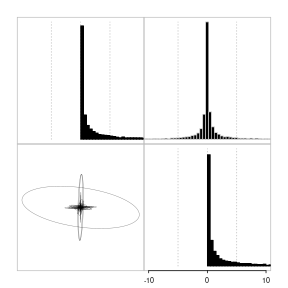Now we can decompose this distribution of variance-covariance matrices in a distribution of correlation matrices and another distribution of standard variations for each trait. For this, we will use the function `samplePrior` to take samples from the prior distribution on the correlation structure and standard deviations separately. One can also use this function to sample the variance-covariance matrices instead of their different components.

``````ss <- samplePrior(n=1000, prior=unif.prior, sample.sd = TRUE, rebuild.R = FALSE)
corr <- sapply(ss\$matrix, function(x) cov2cor(x)[1,2] )
hist( corr, main="Correlation between traits")
hist( ss\$sd[,1], main="Standard deviation - trait 1")
hist( ss\$sd[,2], main="Standard deviation - trait 2")
``````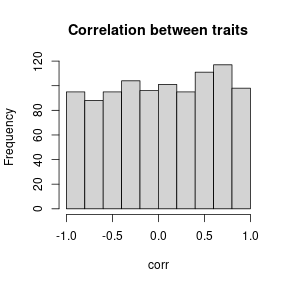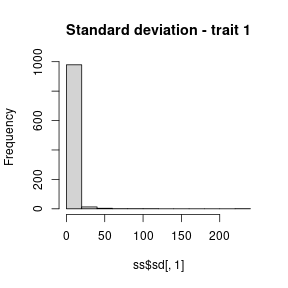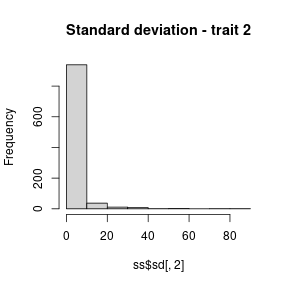Note from these plots that the prior on the correlation is uniformly distributed between -1 and 1. On the other hand, the standard deviations show a log-normal distribution, just as we set it to be. It is difficult to see from the plot of the whole R matrix that the correlations are uniformly distributed, but this is made clearer by transforming the covariance into a correlation.

We already performed the analysis using this data and prior. So we will just plot the posterior distribution for the R matrix. Here note that the red lines show the R matrix used to generate the data.

``````plotRatematrix(chain=post.unif.root, set.leg = c("trait_1", "trait_2")
, point.matrix = list(R), point.color = "red", point.wd = 1.5)
``````
``````## Plotting a single regime.
``````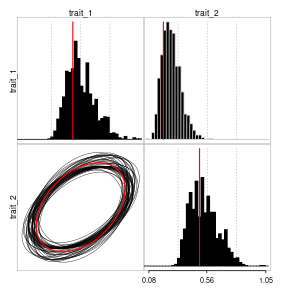Note that the posterior distribution in this case is congruent with the true parameter value used to generated the data.

### Using informative prior distributions on the structure of evolutionary correlation among traits

Here we will repeat the exercise of using a informative prior on the analysis, but now focusing on the structure of evolutionary correlation among traits.

Note that I used a log-normal prior on the standard deviations. Such prior puts more weight toward smaller values for the rates of evolution of the traits. This follows the notion that values for this parameter are usually not very large (and never negative). On the other hand, the marginally uniform prior applied to the correlation matrix sets a uniform prior on the correlation, so negative, positive and no correlation have the same weight (i.e., prior probability).

Maybe we think that this is not a reasonable prior distribution and that strong correlations, either negative or positive, should have less prior probability than weak correlations. For this we will set the prior to be centered on 0 correlation and with most of the density within the -0.5 and 0.5 interval.

Note that here we need to set the option 'unif.corr' to FALSE in order for the function to use the custom input values for the parameters 'Sigma' and 'nu'.

``````## Create a matrix with the bounds for the uniform prior.
## Here will use the max and min of the tip data as bounds.
par.mu <- rbind( ceiling( range(dat[,1]) ), ceiling( range(dat[,2]) ) )
## Using a lognormal prior on the standard deviations, with log(mean)=0 and log(sd)=1.5.
par.sd <- c(0,1.5)
## Sigma is a scale matrix. This is equivalent to the mean of a inverse-Wishart.
## An identity matrix will center the distribution on 0 correlation.
Sigma <- rbind( c(1, 0),
c(0, 1) )
## nu is the equivalent of the variance of this distribution. But here large values mean
##    most of the samples will be close to the Sigma matrix whereas small values
##    (min = number of traits +1) will result in a more spread distribution.
nu <- 50 ## This will constrain the distribution around the Sigma matrix.
corr.prior <- makePrior(r = 2, p = 1, den.mu = "unif", par.mu = par.mu, den.sd = "lnorm"
, par.sd = par.sd, unif.corr = FALSE, Sigma = Sigma, nu = nu)
``````

We can take samples of this prior and make independent plots for the distribution of correlations and standard deviations.

``````ss.corr <- samplePrior(n = 1000, prior=corr.prior, sample.sd = TRUE, rebuild.R = FALSE)
corr.p <- sapply(ss.corr\$matrix, function(x) cov2cor(x)[1,2] )
hist( corr.p, main="Correlation between traits")
hist( ss.corr\$sd[,1], main="Standard deviation - trait 1")
hist( ss.corr\$sd[,2], main="Standard deviation - trait 2")
``````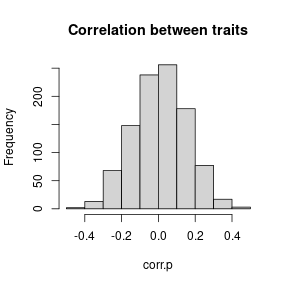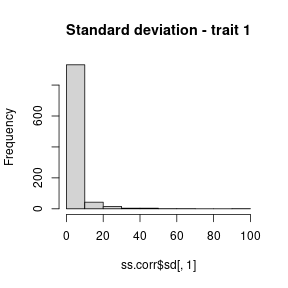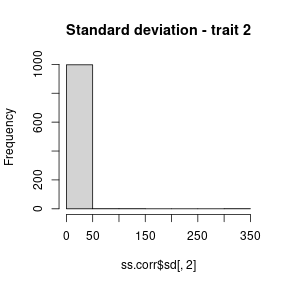Notice how the distribution of standard deviations has not changed even after modifying the prior on the correlation. This allows for great flexibility for setting prior distributions when using the `ratematrix` package. Note that we successfully set the prior on the correlations as intended (nice!).

The chosen prior is very informative and is does not span the true parameter value for the simulation. The true correlation value used in the simulations is:

``````cov2cor(R)[1,2]
``````
``````##  0.4
``````

After sampling from the prior distribution using `samplePrior` we can apply the function `plotRatematrix` to make a plot of the distribution of R matrices. The function `plotratematrix` will rebuild the variance-covariance matrices from the joint distribution of correlation matrices and standard deviations prior to making the plot. This is possible because `samplePrior` produces output in the same format as the `readMCMC` function.

``````plotRatematrix(ss.corr, set.xlim = c(-10,10), show.zero = TRUE)
``````
``````## Plotting a single regime.
``````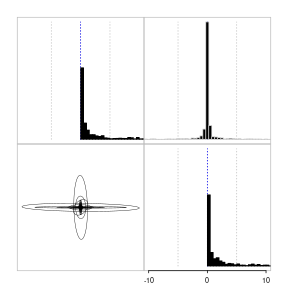We can compare this prior distribution with the prior distribution used in the previous analysis. It is possible to see how the covariance term is much more tightly distributed around 0.

Now we can set and run the analysis using this prior, then check convergence and plot the posterior distribution.

``````## handle.corr.zero <- ratematrixMCMC(data=dat, phy=phy, prior=corr.prior
##                                    , start="prior_sample", gen=200000
##                                    , outname="corr_zero_prior", prop=c(0.1,0.45,0.45)
##                                    , dir=tempdir() )
checkConvergence(post.corr.zero)
``````
``````## \$heidel
##       root matrix
## stest TRUE   TRUE
## htest TRUE   TRUE
##
## \$ess
##       root_1       root_2 matrix_cel_1 matrix_cel_2 matrix_cel_3 matrix_cel_4
##     141.6506     112.1025     692.3728     734.7567     734.7567     689.4026
``````
``````plotRatematrix(post.corr.zero, point.matrix = list(R), point.color = "red", point.wd = 1.5
, show.zero = TRUE)
``````
``````## Plotting a single regime.
``````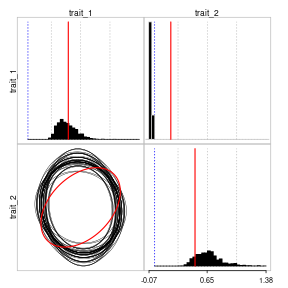The result is similar to when we set an informative prior to the vector of root values. The posterior distribution for the evolutionary covariance among traits is not centered in the true parameter value whereas it was in the previous analysis with the marginally uniform prior. The bias is also predictable; the posterior distribution has shifted towards the zero value.

## Final considerations

It is important to note that in empirical studies there is no such thing as “true parameter values”. While it is straightforward to show the impact of the prior distribution using simulations, it is much harder with empirical data. For this reason, we recommend that users actively explore different prior distributions. Also, a Maximum Likelihood Estimate (MLE) can be performed with packages such as `mvMORPH` and `phytools`. The MLE result should be compared with the Bayesian posterior distribution. If the MLE is not congruent with the MCMC analysis, some questions need to be answered before moving forward; does the MLE reached a global solution? Is the prior distribution away from the MLE? Have the MCMC really converged?

“And a lean, silent figure slowly fades into the gathering darkness, aware at last that in this world, with great power there must also come – great responsibility!” (Stan Lee and Steve Ditko, 1962. Amazing Fantasy #15)